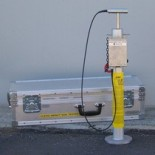Impact Soil TesterHome Contact Newsletter 14                       January 1995 (rev 8/8/99) CLEGG HAMMER MODULUS (CHM) There has been increasing interest in using the Clegg Impact Soil Tester (CIST) as a means of arriving at a design elastic modulus for application to such problems as multi-layer analysis of soil structures, particularly in the road pavement context. The use of such computer programs as CIRCLY, ELMOD, EFROMD, etc. involving the use of an elastic modulus either as a Resilient Modulus from a test measurement or an Equivalent Elastic Modulus for in situ material determined by "back calculation" involving the use of a "seed" modulus for iterative analysis has encouraged the further analysis of the relationship between Clegg Impact Value (CIV) and modulus. Conversion from CIV to Clegg Hammer Modulus (CHM) can be made by applying theoretical concepts by which to produce relationships for the commonly used Hammers * (refer to Technical Notes TN 1 & TN 2 regarding the theory). The basic equations are tabulated as follows: 20 kg, 30 cm drop, 13 cm diameter: (CHM/H) MPa = 0.23 (CIV/H)2 4.5 kg, 45 cm drop, 5 cm diameter: (CHM/S) MPa = 0.088 (CIV)2 2.25 kg, 45 cm drop, 5 cm diameter: (CHM/M) MPa = 0.044 (CIV/M)2 0.5 kg, 30 cm drop, 5 cm diameter: (CHM/L) MPa = 0.015 (CIV/L)2 The coefficients in these equations have been derived using double integration of time vs. deceleration to determine the deflection and using this in elastic plate bearing theory to arrive at an elastic modulus. They depend to some extent on the technique used for the integration and the theoretical assumptions. The use of CHM as a "seed" modulus for iterative analysis comparing calculated deflections with field observations should enable the coefficients to be refined from time to time. Because of the relative simplicity of the test for CIV and the fact that it can be performed both in the laboratory and field, there are cost as well as technical advantages and the technique suggested herein appears worthy of consideration. For further discussion or information on this matter, please contact us as per our details below. * The 20 kg CIST is called the "Heavy" CIST and hence CIV/H and CHM/H to denote the CIV and the CHM of this mass with its diameter and drop height. Likewise, the 2.25 kg CIST is known as the "Medium" Hammer and so CIV/M and CHM/M. The 0.5 kg CIST is called the "Light" CIST so the notation is seen as CIV/L and CHM/L. The 4.5 kg CIST is considered the "Standard" CIST. CIV using the Standard CIST is usually notated simply as CIV but CHM/S is useful so as to distinguish a Clegg Hammer Modulus derived using the Standard CIST.

Home ]